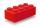# Volume + right triangle - examples

1. Prism 4 sidesThe prism has a square base with a side length of 3 cm. The diagonal of the sidewall of the prism/BG/is 5 cm. Calculate the surface of this prism in cm square and the volume in liters
2. The diagram 2The diagram shows a cone with slant height 10.5cm. If the curved surface area of the cone is 115.5 cm2. Calculate correct to 3 significant figures: *Base Radius *Height *Volume of the cone
3. Pile of sandA large pile of sand has been dumped into a conical pile in a warehouse. The slant height of the pile is 20 feet. The diameter of the base of the sand pile is 31 feet. Find the volume of the pile of sand.
4. Axial sectionAxial section of the cone is equilateral triangle with area 208 dm2. Calculate volume of the cone.
5. CuboidCuboid with edge a=16 cm and body diagonal u=45 cm has volume V=11840 cm3. Calculate the length of the other edges.
6. CubesOne cube is inscribed sphere and the other one described. Calculate difference of volumes of cubes, if the difference of surfaces in 257 mm2.
7. Cuboid diagonalCalculate the volume and surface area of the cuboid ABCDEFGH, which sides abc has dimensions in the ratio of 9:3:8 and if you know that the wall diagonal AC is 86 cm and angle between AC and the body diagonal AG is 25 degrees.
8. Circular poolThe base of pool is circle with a radius r = 10 m excluding circular segment that determines chord length 10 meters. Pool depth is h = 2m. How many hectoliters of water can fit into the pool?
9. Floating barrelBarrel (cylinder shape) floats on water, top of barrel is 8 dm above water and the width of surfaced barrel part is 23 dm. Barrel length is 24 dm. Calculate the volume of the barrel.
10. Tetrahedral pyramidCalculate the volume and surface area of a regular tetrahedral pyramid, its height is \$b cm and the length of the edges of the base is 6 cm.
11. Triangular prismPlane passing through the edge AB and the center of segmet CC' of regular triangular prism ABCA'B'C', has angle with base 22 degrees, |AB| = 6 cm. Calculate the volume of the prism.
12. 4side pyramidCalculate the volume and surface of 4 side regular pyramid whose base edge is 4 cm long. The angle from the plane of the side wall and base plane is 60 degrees.
13. Tetrahedral pyramidCalculate the volume and surface of the regular tetrahedral pyramid if content area of the base is 20 cm2 and deviation angle of the side edges from the plane of the base is 60 degrees.
14. Triangular pyramidCalculate the volume and surface area of a regular triangular pyramid whose height is equal to the length of the base edges 10 cm.
15. Hexagonal pyramidCalculate the volume and the surface of a regular hexagonal pyramid with a base edge length 3 cm and a height 5 cm.
16. Two ballsTwo balls, one 8cm in radius and the other 6cm in radius, are placed in a cylindrical plastic container 10cm in radius. Find the volume of water necessary to cover them.
17. BlockCalculate the volume of a cuboid ABCDEFGH if |AB| = 16 cm, |BC| = 19 cm and the angle ∠CDG = 36.9°
18. Hexagonal prismThe base of the prism is a regular hexagon consisting of six triangles with side a = 12 cm and height va = 10.4 cm. The prism height is 5 cm. Calculate the volume and surface of the prism!
19. Triangular prismCalculate the surface area and volume of a triangular prism, base right triangle if a = 3 cm, b = 4 cm, c = 5 cm and height of prism h=12 cm.
20. Triangular prismBase of perpendicular triangular prism is a right triangle with leg length 5 cm. Content area of the largest side wall of its surface is 130 cm² and the height of the body is 10 cm. Calculate its volume.

Do you have an interesting mathematical example that you can't solve it? Enter it, and we can try to solve it.

To this e-mail address, we will reply solution; solved examples are also published here. Please enter e-mail correctly and check whether you don't have a full mailbox.

Tip: Our volume units converter will help you with converion of volume units. See also our right triangle calculator. See also more information on Wikipedia.